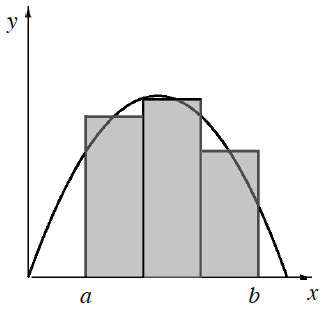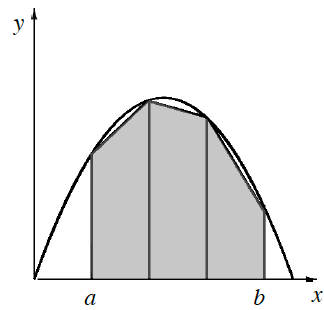### Home > APCALC > Chapter 1 > Lesson 1.3.2 > Problem1-121

1-121.

WHICH IS BETTER? Part Two

Below is a comparison between using rectangles and trapezoids to approximate the area under a curve for the same interval of a function. Decide which method you think will best approximate the area under the curve for $a ≤ x ≤ b$. Then approximate the area using each method if $f(x) = -0.25x(x - 9)$, $a = 2$, and $b = 8$ using $3$ sections. Compare your results with the actual area $A = 25.5 \text{ un}^2$.Midpoint RectanglesTrapezoids

Which method comes closer to the actual curve $f(x)$?

Each section is $2$ units wide. Use this knowledge and the equation of the function to find the areas of each section for both methods.

Which method gave you the number closest to $25.5$?